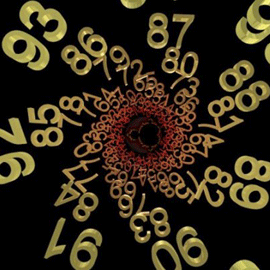## The Destiny Numbers in NumerologyMost people are unaware of the fact that their birth name holds a lot of significance in numerology systems. When beginning in numerology you will want to take the time to fully understand the basics of this science. With numerology having existed on our planet for thousands of years, the numerous methods of calculating your numbers can become overwhelming.

The destiny number is a number that you will want to calculate from the start. This number is often referred to as the expression number as well which can often cause some confusion with people who are new to numerology. Calculating the destiny or expression number is simple and can be perform with very little knowledge about numerology. Being one of the easiest calculations to make in numerology, the destiny number is often one of the first that people tend to calculate.

Unlike the life path number or the birthday number, the destiny number does not use the date of birth of an individual in order to determine that person’s fate. Instead, the destiny number uses the full name of an individual in order to make the calculation. In order for this to be possible, you need to convert all of the letters of your full name into single digit numbers.

Calculating the numbers in your name requires you to know which letter is represented by which number. This is often what becomes too complicated for some people to understand, yet it is an extremely simple to grasp notion of numerology. The numbers cannot go over 9 so each letter is represented by a number that ranges from 1 to 9.

In order to calculate the value of a letter you should probably take a piece of paper and write down each letter of the alphabet. Then starting with the letter A give each following letter a higher number until you reach 9. Once you reach the number 9 you have to give the following letter the number 1 and start over.

For example, the first few letters would look like this; A-1, B-2, C-3, D-4, E-5, F-6, G-7, H-8, I-9, J-1, K-2 and so on until you reach the letter Z. Once you have figured out which number represent each letter you will want to write down the numbers that represent each letter in your name. In order to calculate the destiny number you will need to add all of these numbers together.

It’s important to remember that numbers are almost always reduced to single digits in numerology so your results will have to also be reduced to single digits. Let’s say your name is David Johnson, the letters of your name would be the following: 4, 1, 4, 9, 4, 1, 6, 8, 5, 1, 6, 5. It’s important to add each name separately, reduce each name to single digits and then add the two names together and once again reduce it to single digits.

In this case, the two names would equal the numbers 22 and 32. When reducing them to single digits they equal 4 and 5 and then when adding them together you get 9. So if your name was David Johnson, your destiny number would be 9.